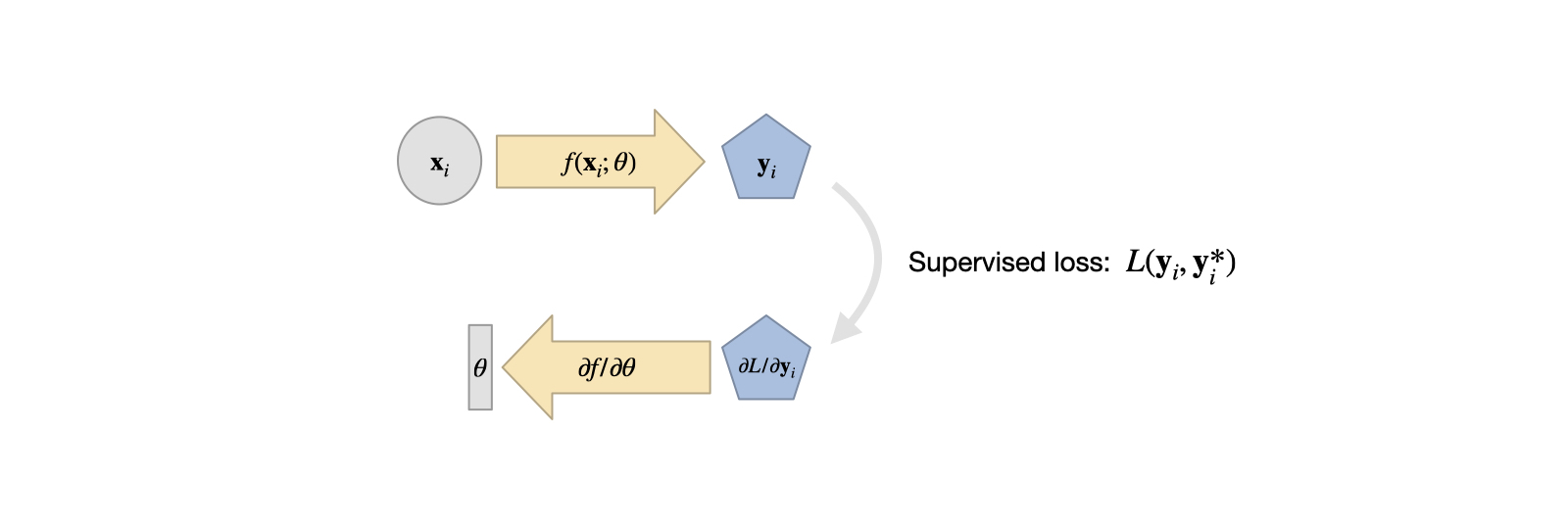# Supervised Training#

Supervised here essentially means: “doing things the old fashioned way”. Old fashioned in the context of deep learning (DL), of course, so it’s still fairly new. Also, “old fashioned” doesn’t always mean bad - it’s just that later on we’ll discuss ways to train networks that clearly outperform approaches using supervised training.

Nonetheless, “supervised training” is a starting point for all projects one would encounter in the context of DL, and hence it is worth studying. Also, while it typically yields inferior results to approaches that more tightly couple with physics, it can be the only choice in certain application scenarios where no good model equations exist.

## Problem setting#

For supervised training, we’re faced with an unknown function $$f^*(x)=y^*$$, collect lots of pairs of data $$[x_0,y^*_0], ...[x_n,y^*_n]$$ (the training data set) and directly train an NN to represent an approximation of $$f^*$$ denoted as $$f$$.

The $$f$$ we can obtain in this way is typically not exact, but instead we obtain it via a minimization problem: by adjusting the weights $$\theta$$ of our NN representation of $$f$$ such that

(15)#$\text{arg min}_{\theta} \sum_i \Big(f(x_i ; \theta)-y^*_i \Big)^2 .$

This will give us $$\theta$$ such that $$f(x;\theta) = y \approx y^*$$ as accurately as possible given our choice of $$f$$ and the hyperparameters for training. Note that above we’ve assumed the simplest case of an $$L^2$$ loss. A more general version would use an error metric $$e(x,y)$$ in the loss $$L$$ to be minimized via $$\text{arg min}_{\theta} \sum_i e( f(x_i ; \theta) , y^*_i) )$$. The choice of a suitable metric is a topic we will get back to later on.

Irrespective of our choice of metric, this formulation gives the actual “learning” process for a supervised approach.

The training data typically needs to be of substantial size, and hence it is attractive to use numerical simulations solving a physical model $$\mathcal{P}$$ to produce a large number of reliable input-output pairs for training. This means that the training process uses a set of model equations, and approximates them numerically, in order to train the NN representation $$f$$. This has quite a few advantages, e.g., we don’t have measurement noise of real-world devices and we don’t need manual labour to annotate a large number of samples to get training data.

On the other hand, this approach inherits the common challenges of replacing experiments with simulations: first, we need to ensure the chosen model has enough power to predict the behavior of real-world phenomena that we’re interested in. In addition, the numerical approximations have numerical errors which need to be kept small enough for a chosen application. As these topics are studied in depth for classical simulations, and the existing knowledge can likewise be leveraged to set up DL training tasks.Fig. 5 A visual overview of supervised training. Quite simple overall, but it’s good to keep this in mind in comparison to the more complex variants we’ll encounter later on.#

## Surrogate models#

One of the central advantages of the supervised approach above is that we obtain a surrogate model, i.e., a new function that mimics the behavior of the original $$\mathcal{P}$$. The numerical approximations of PDE models for real world phenomena are often very expensive to compute. A trained NN on the other hand incurs a constant cost per evaluation, and is typically trivial to evaluate on specialized hardware such as GPUs or NN units.

Despite this, it’s important to be careful: NNs can quickly generate huge numbers of in between results. Consider a CNN layer with $$128$$ features. If we apply it to an input of $$128^2$$, i.e. ca. 16k cells, we get $$128^3$$ intermediate values. That’s more than 2 million. All these values at least need to be momentarily stored in memory, and processed by the next layer.

Nonetheless, replacing complex and expensive solvers with fast, learned approximations is a very attractive and interesting direction.

## Show me some code!#

Let’s finally look at a code example that trains a neural network: we’ll replace a full solver for turbulent flows around airfoils with a surrogate model from [TWPH20].